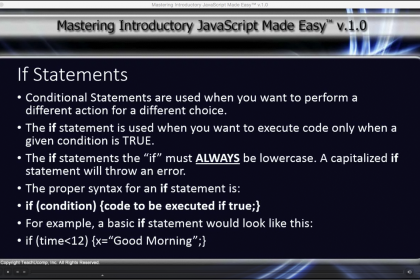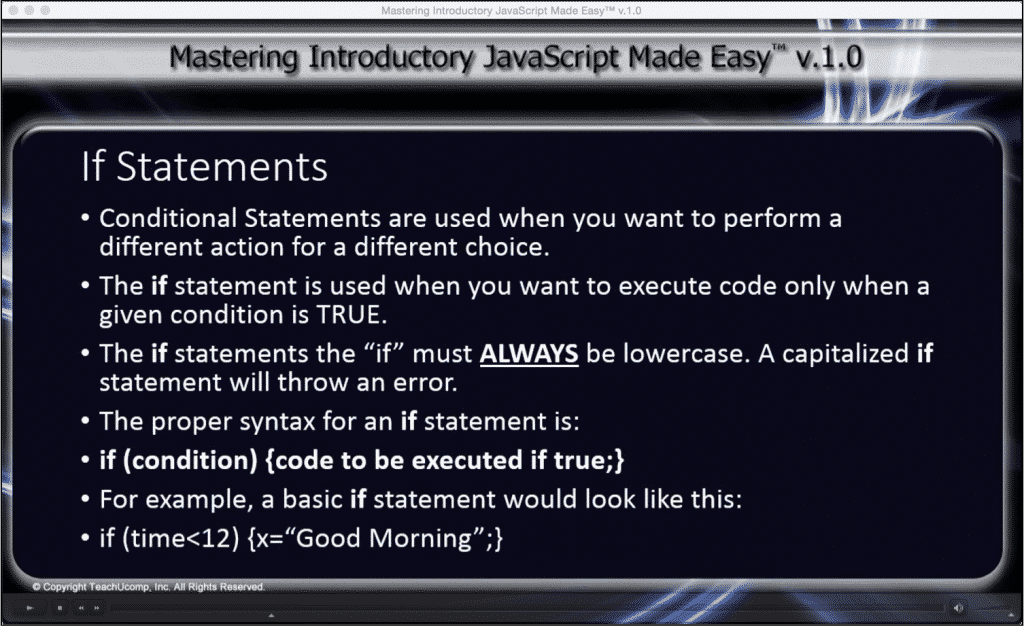# If Statement in JavaScript- A Tutorial

/ / JavaScript, Latest## If Statement in JavaScript: Overview

In JavaScript, conditional statements are used when you want to perform different actions for different choices. The if statement in JavaScript is used when you want to execute code only when a given condition is true. When using any if statement in JavaScript it is important to remember to NOT capitalize the “if.” A capitalized “if” will return a JavaScript error. The syntax for an if statement in JavaScript is:

if (condition) {code to be executed if condition is true;}

The if…else statement is used when you want to execute some code when the condition is true and some other code if the condition is false. The syntax for an if…else statement is:

if (condition) {code to be executed if condition is true;}
else {code to be executed if condition is not true;}

There is also the if…else if…else statement. This statement is used when you want to execute one of several blocks of code. The syntax for an if…else if…else statement is:

if (condition1) {code to be executed if condition1 is true;}
else if (condition2) {code to be executed if condition2 is true;}
else {code to be executed if neither condition1 nor condition2 is true;}

 Example 1: if (time<12) {x=”Good Morning“;} Result: Sets the if condition to less than 12pm. (JavaScript uses a 24 hour clock) Gives x the value of “Good Morning”. When executed, if time is less than 12pm, will display “Good Morning”. If time is greater than 12pm no information will be displayed. Example 2: if (time<17) {x=”Good Day“;} else {x=”Good Evening“;} Result: Sets the if condition to less than 5pm. If time is less than 5pm when executed will display “Good Day”. If time is greater than 5pm when executed will display “Good Evening”. Example 3: if (time<12) {x=”Good Morning“;} else if (time<17) {x=”Good Day“;} else {x=”Good Evening“;} Result: Sets first if condition to less than 12pm and if true displays “Good Morning”. Sets else and second if condition to less than 5pm and if true will display “Good Day”. Sets second else condition to display “Good Evening” if previous conditions are false.## If Statement in JavaScript: Instructions

1. Type: if (a)

{b…;}

Where “a” is the condition to be met and “b” is the code to be executed if the condition is true.

2. Type:  if (a)

{b…;}

else

{c…;}

Where “a” is the condition to be met, “b” is the code to be executed if the condition is true, and “c” is the code to be executed if the condition is not true.

3. Type: if(a)

{b…;}

else if (c)

{d…;}

else

{e…;}

Where “a” is the first condition to be met, “b” is the code to be executed if the first condition is true, “c” is the second condition, “d” is the code to be executed if the second condition is true, and “e” is the code to be executed if neither the first nor second condition are true.

## If Statement in JavaScript: Instructions

The following video lesson, titled “9.1- If Statements,” discusses the syntax of the If statement in JavaScript. This lesson is from our Introductory JavaScript tutorial, titled “Mastering Introductory JavaScript Made Easy v.1.0.”

TOP
Sale! \$49 All-Access 2 Days 8 Hours 23 Minutes 15 Seconds      \$199 \$49 Entire Library!